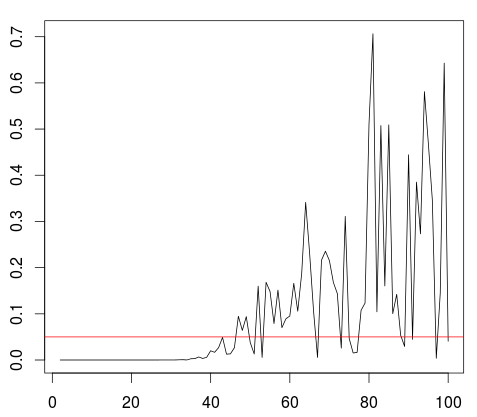Want to share your content on R-bloggers? click here if you have a blog, or here if you don't.

The idea of GLMs is that given some covariates has a distribution in the exponential family (Gaussian, Poisson, Gamma, etc). But that does not mean that  has a similar distribution… so there is no reason to test for a Gamma model for  before running a Gamma regression, for instance. But are there cases where it might work? That the non-conditional distribution is the same (same family at least) than the conditional ones?

For instance, if  has a joint Gaussien distribution, then both marginals are Gaussian, but also . So, in that case, if the covariate is normally distributed, it is possible to have a Gaussian distribution also for . The econometric interpretation is that with a standard Gaussian linear model, if is normally distributed, not only the conditional distribution  is Gaussian but also the non-conditional distribution of .

```> set.seed(1)
> n=1e3
> X=rnorm(n,10,2)
> Y=1+3*X+rnorm(n)
> plot(X,Y,xlim=c(4,20))```Indeed, here the distribution of  is also Gaussian

```> library(nortest)

Anderson-Darling normality test

data:  Y
A = 0.23155, p-value = 0.802

> shapiro.test(Y)

Shapiro-Wilk normality test

data:  Y
W = 0.99892, p-value = 0.8293```

(not only from a statistical point of view, the thoery of Gaussian random vectors confirms that the non-conditional distribution is Gaussian actually)Here  is continuous. What if we consider a finite mixture here, i.e. takes only a finite number of values? Actually, Teicher (1963) proved that it is not possible to have a non-conditional Gaussian distribution for . But in practice, would we really reject the Gaussian assumption, for ? If the number of classes is to small, yes. But with a large number of classes (a sufficiently large number of mixture components), it is possible,

```> pv=function(k=2){
+ n=1e4
+ X=rnorm(n,10,2)
+ Q=quantile(X,(0:k)/k)
+ Q=0
+ Xc=cut(X,Q,labels=1:k)
+ XcN=tapply(X,Xc,mean)
+ Xn=XcN[as.numeric(Xc)]
+ Y=1+3*Xn+rnorm(n)

> plot(2:100,Vectorize(pv)(2:100),type="l")
> abline(h=.05,col="red")```So here, it could be possible to have also a Gaussian distribution, for . As least to accept that assumption, statistically.

In the context of a Poisson regression, it is well know that it’s not possible to have at the same time  that is Poisson distributed (that’s a Poisson regression) and also  that is Poisson distributed. That simply comes from the fact that

while

and because of the conditional Poisson distribution, then

Thus,

So  cannot be Poisson distribution. But again, it could be possible, if heterogeneity is not too large, to accept the null assumption of a Poisson distribution for .

More generally, it is very difficult to have a distribution family for   that is also the distribution of the non-conditional variable . In the context of a finite mixture ( takes a finite number of values),Teicher (1963) proved that it was not not possible, neither for the Gaussian distribution nor the Gamma distribution. An to go further, check Monfrini (2002) (thanks Romuald for point out the reference).

Hence, as a keep saying, before running a regression model on with some given family, it is never a good idea to check if the non-conditional distribution  has the same distribution. Because there is no reason, usually, to remain in the same family.